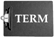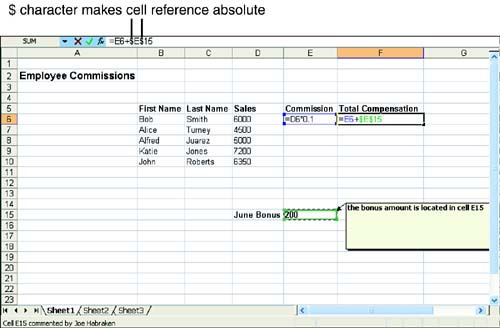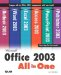# Using Relative and Absolute Cell Addresses

### Using Relative and Absolute Cell Addresses

As mentioned at the beginning of this lesson, when you copy a formula from one place in the worksheet to another, Excel adjusts the cell references in the formulas relative to their new positions in the worksheet. There might be occasions when you don't want Excel to change the reference related to a particular cell that appears in a formula (or in an Excel function, which is discussed in Lesson 5, "Performing Calculations with Functions").Absolute Versus Relative An absolute reference is a cell reference in a formula that does not change when copied to a new location; you designate it as absolute. A relative reference is a cell reference in a formula that is adjusted when the formula is copied.

For example, suppose you have a worksheet that computes the commission made on sales by each of your salespeople. Sales have been so good that you've decided to give each person on the sales team a \$200 bonus. Figure 4.2 shows the worksheet that you've created.

##### Figure 4.2. Some formulas require absolute references.Notice that the bonus amount is contained in only one cell on the worksheet (cell E15). Therefore, when you create the formula used in F6 and then copied to cells F7 through F11, you need to make sure that the bonus amount in cell E15 is always referenced by the formula. This is a case where you must " absolutely " reference the bonus amount in cell E15.

To make a cell reference in a formula absolute, add a \$ (dollar sign) before the column letter and before the row number that make up the cell address. For example, in Figure 4.2, the formula in F6 must read as follows :

` =E6+\$E `

The address, \$E\$15, refers to cell E15, meaning that cell E15 is absolutely referenced by the formula. This cell reference remains "locked" even when you copy the formula to the other cells in the E column.

To create an absolute reference in a formula (or a function, which is discussed in Lesson 5), create your formula as you normally would (as detailed in Lesson 3, "Performing Simple Calculations"). After typing or pointing out a cell address in a formula that needs to be an absolute reference, press F4 . A dollar sign (\$) is placed before the cell and row designation for that cell. Once data is labeled as absolute, you will find that if you move the data to a different cell (or cells), the formula will update itself to reference the new location.

Some formulas might contain cell addresses where you will make the column designation absolute, but not the row (or vice versa). For example, you could have a formula \$A6/2. You are telling Excel that the values will always be contained in column A (it is absolute), but the row reference (6) can change when the formula is copied. Having a cell address in a formula that contains an absolute designation and a relative reference is called a mixed reference .Mixed References A reference that is only partially absolute, such as A\$2 or \$A2. When a formula that uses a mixed reference is copied to another cell, only part of the cell reference (the relative part) is adjusted.

Absolute referencing and mixed references are also required by some of Excel's built-in functions. You work with functions in the next lesson.Microsoft Office 2003 All-in-One
ISBN: B005HKSHB2
EAN: N/A
Year: 2002
Pages: 660
Authors: Joe Habraken

Similar book on Amazon